# VBA Course: Properties

In this exercise, we will write VBA code that modifies the content and appearance of cells and worksheets.

First open the editor, add a module, copy the macro below into it, and link it to a formula button (refer back to the previous page if needed):

`Sub properties()    'Incomplete Macro    Range ("A8")End Sub`

We want to modify cell A8 at the beginning of this macro.

To display the list of possible things that can be associated with the Range object, add a period after Range("A8"):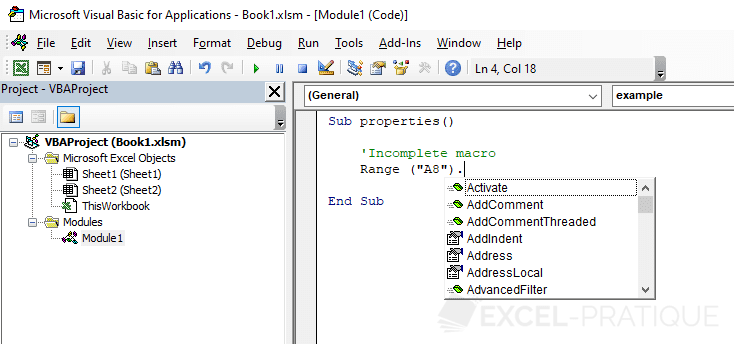The editor will now display the various possibilities ...

In this first example, click on "Value" and then on the Tab key to validate your choice.

`Sub properties()    'Incomplete Macro    Range("A8").ValueEnd Sub`

In this case, the property, Value, represents the contents of the cell.

Next, we will assign the value 48 to cell A8:

`Sub properties()    'A8 = 48    Range("A8").Value = 48    'Translation:    'The value of cell A8 is equal to 48End Sub`

Then we will assign the value Sample text to cell A8 (important: the text must be within " "):

`Sub properties()    'A8 = Sample text    Range("A8").Value = "Sample text"End Sub`

In this case, we're going to modify cell A8 on the worksheet, the cell from which the procedure is actually launched (using a formula button). If you create a second button like this on worksheet 2, it will modify cell A8 on that sheet (sheet 2).

To make it modify cell A8 on sheet 2 when you click the button on sheet 1, you have to add the following before Range: Sheets("Name_of_the_sheet") or Sheets(Number_of_the_sheet).

`Sub properties()    'A8 on sheet 2 = Sample text    Sheets("Sheet2").Range("A8").Value = "Sample text"    'Or:    'Sheets(2).Range("A8").Value = "Sample text"End Sub`

Just the same, if we wanted to modify cell A8 on sheet 2 of another open workbook, we have to add the following before Sheets and Range: Workbooks("Name_of_the_file").

`Sub properties()    'A8 on sheet 2 of workbork 2 = Sample text    Workbooks("Book2.xlsx").Sheets("Sheet2").Range("A8").Value = "Sample text"End Sub`

Although we used Value in these examples, you don't really need to use it, because if nothing else is specified, it will be the value of the cell that is modified.

For example, these two lines would have the same effect:

`Range("A8").Value = 48Range("A8") = 48`

## Erase cell contents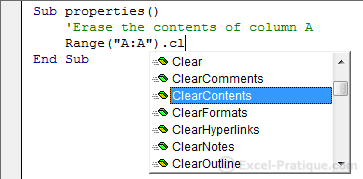`Sub properties()    'Erase the contents of column A    Range("A:A").ClearContentsEnd Sub`

## Text Formatting

When you open Font., the list of properties that can be applied to text formatting will appear: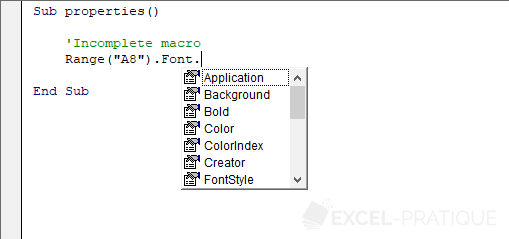We'll explain in detail how to edit the colors on the next page ...

## Formatting: change text size

`Sub properties()    'Edit the size of text in cells A1 through A8    Range("A1:A8").Font.Size = 18End Sub`

## Formatting: make text bold

`Sub properties()    'Make cells A1 through A8 bold    Range("A1:A8").Font.Bold = TrueEnd Sub`

Bold = True means Characters will appear in bold = Yes.

To remove the "bold" formatting from text, all you have to do is replace "Yes" with "No", or in other words, "True" with "False":

`Sub properties()    'Remove "bold" formatting from cells A1 through A8    Range("A1:A8").Font.Bold = FalseEnd Sub`

## Formatting: italicize text

`Sub properties()    'Italicize cells A1 through A8    Range("A1:A8").Font.Italic = TrueEnd Sub`

## Formatting: underline text

`Sub properties()    'Underline cells A1 through A8    Range("A1:A8").Font.Underline = TrueEnd Sub`

## Formatting: Set font

`Sub properties()    'Edit font in cells A1 through A8    Range("A1:A8").Font.Name = "Arial"End Sub`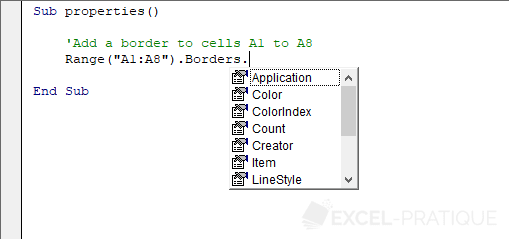`Sub properties()    'Add a border to cells A1 to A8    Range("A1:A8").Borders.Value = 1    'Value = 0    => no borderEnd Sub`

## Change the formatting of currently selected cells

`Sub properties()    'Add a border to selected cells    Selection.Borders.Value = 1End Sub`

## Change a worksheet's properties

`Sub properties()    'Hide a worksheet    Sheets("Sheet3").Visible = 0    'Visible = -1     => cancels the effectEnd Sub`
Don't forget that we've only introduced a tiny minority of the possible customizations that can be done with VBA.
If the property that you are looking for isn't described in detail here, don't be afraid to look for it in the list of properties in Excel or in Excel's own help files.
The macro recorder can also save you a lot of time if you don't know the name of a property. If you record the action that you need, it will be easy to find the name of the property so that you can then use it in your own macro.

## Change the value of a cell based on another cell

In this case, we want A7 to take its value from A1: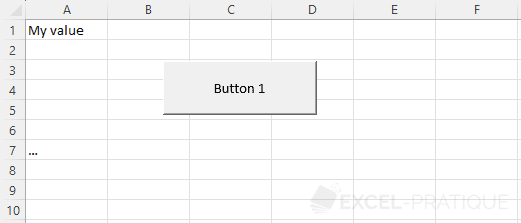So we will tell A7 to take its value from A1, which would look like this:

`Sub properties()    'A7 = A1    Range("A7") = Range("A1")    'Or:    'Range("A7").Value = Range("A1").ValueEnd Sub`

If we only wanted to copy the text size from the other cell, the code would look like this:

`Sub properties()    Range("A7").Font.Size = Range("A1").Font.SizeEnd Sub`
Anything on the left side of the = takes on the value of what is on the right side of the =.

## Change the value of a cell based on its own value

Now we're going to create a click counter.

Each time we click, the value of A1 will be incremented by 1:

`Sub properties()    'Click counter in A1    Range("A1") = Range("A1") + 1End Sub`

Excel executes the code line by line, so these commentaries should help you understand the code itself:

`'For example: before the code is executed, A1 has the value 0Sub properties()    'The button has been clicked, so the procedure is starting    'For the moment, A1 still has the value 0        'DURING the execution of the line immediately below, A1 still has the value 0    Range("A1") = Range("A1") + 1 'And now the calculation is: New_value_of_A1 = 0 + 1    'A1 has the value 1 only AFTER the execution of the line of code    End Sub`

## With

This code makes it possible to set different properties of the active cell:

`Sub properties()    ActiveCell.Borders.Weight = 3    ActiveCell.Font.Bold = True    ActiveCell.Font.Size = 18    ActiveCell.Font.Italic = True    ActiveCell.Font.Name = "Arial"End Sub`

In this case, we can use With to avoid having to repeat ActiveCell.

Now you will see how With works:

`Sub properties()    'Beginning of instructions using command: WITH    With ActiveCell        .Borders.Weight = 3        .Font.Bold = True        .Font.Size = 18        .Font.Italic = True        .Font.Name = "Arial"    'End of instructions using command: END WITH    End WithEnd Sub`

This way we don't have to repeat ActiveCell.

Although it isn't really necessary in this case, we could avoid repeating .Font, too, which would look like this:

`Sub properties()    With ActiveCell        .Borders.Weight = 3        With .Font            .Bold = True            .Size = 18            .Italic = True            .Name = "Arial"        End With    End WithEnd Sub`